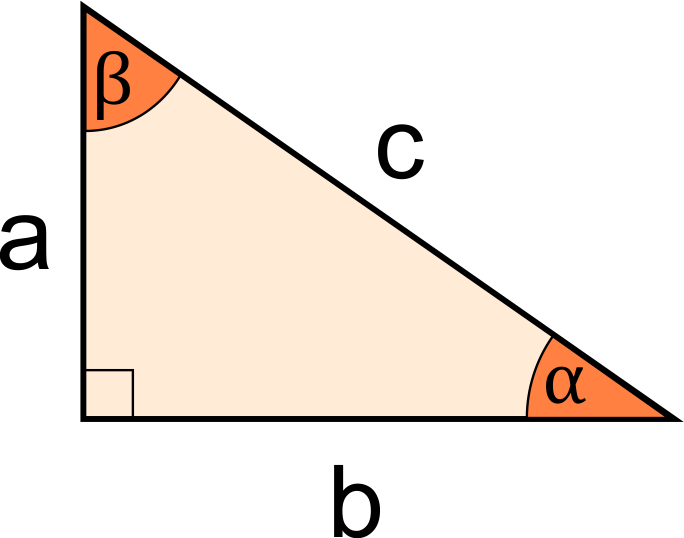# Similar Right Triangles Calculator

Reviewed by Rahul Dhari
Last updated: Jun 27, 2022

Our similar right triangles calculator helps you calculate the missing side or angle when two right triangles are given to be similar. Read on to know more about what similar right triangles mean, what scale factor refers to, and also how to find the missing measurements in two given similar right triangles.

## What are similar right triangles?

Two right triangles are similar if:

• They both have the same set of 3 angles; and
• Their sides are proportional.

The ratio of the lengths of corresponding sides of these triangles is called the scale factor.

## How do I use the similar right triangles calculator?

To use this tool for finding missing sides or angles in similar right triangles, you need to know at least 2 sides of the first triangle. Additionally, you need to know at least one of the following:

• The scale factor; or
• At least 2 sides of the second triangle.

The steps to use this similar right triangles calculator are as follows:

1. Enter the side lengths of at least 2 sides in the first right triangle;
2. If you know the scale factor, enter its value. Voila! Using this, the tool will display all side and angle measurements for the second triangle!
3. Alternatively, if the scale factor is not known, enter the measurements of at least 2 sides of the second triangle. The tool will show you all the unknown angle and area measurements!

## Other similarity calculators

If you found this similar right triangles calculator useful, you may also want to check out some of our other similarity tools, listed below:

• Similar triangles calculator;
• Solve similar triangles calculator; and
• Check similarity in right triangles calculator.

## FAQ

### Does 5/12/13 make a right triangle?

Yes! 5 ,12 and 13 make a right triangle. These set of numbers are sometimes referred to as Pythagorean triplets, since they satisfy the Pythagorean theorem as follows:

1. The square of 5 is 25;
2. The square of 12 is 144;
3. The sum of 25 and 144 is 169, which is equal to the square of 13!

### How do you find similar right triangles?

To find similarity in right triangles, one of these conditions must be satisfied:

• All 3 angle measures must be the same in both triangles;
• Corresponding pairs of legs must be proportional in both right triangles; or
• The ratio of one pair of corresponding legs must be equal to the ratio of the 2 hypotenuses taken in the same order.
First triangle measurements (Enter any 2 sides)Length of a (leg)
in
Length of b (leg)
in
Length of c (hypotenuse)
in
Scale factor (Side length in △₂ / Side length in △₁)
Is the scale factor known?
yes
Scale factor
People also viewed…

### Complex conjugate

The complex conjugate calculator is here to become your favorite tool to find the complex conjugate of a number.

### Percentage of a percentage

Percentage of percentage allows you to multiply % by % and get the result.

### Plastic Footprint

Find out how much plastic you use throughout a year with this plastic footprint calculator. Rethink your habits, reduce your plastic waste, and make your life a little greener.

### Titration

Use our titration calculator to determine the molarity of your solution.